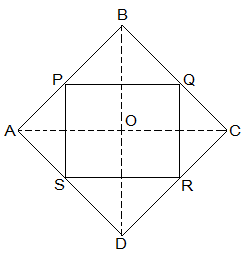# ABCD is a rhombus and P, Q, R and S are the mid-points of the sides AB, BC, CD and DA respectively. Show that the quadrilateral PQRS is a rectangle.Considering ∆ABD

We observe that P and S are the mid points of side AB and AD respectively. [Given]

We know the theorem: The line segment joining the mid-points of two sides of a triangle is parallel to the third side

Hence, PS || BD and PS = ½ BD (From above theorem)……………………….(1)

Similarly, is we take ∆CBD

We observe that R and Q are the mid points of side CD and CB respectively. [Given]

Hence, RQ || BD and RQ = ½ BD (From above theorem)……………………….(2)

So from (1) and (2), we conclude that

PS || RQ and PS = RQ

If each pair of opposite sides of a quadrilateral is equal, then it is a parallelogram.

Hence, PQRS is a parallelogram.

Now in ∆ACD

We observe that S and R are the mid points of side AD and CD respectively. [Given]

Hence, SR || AC and RS = ½ AC (From above theorem)

from (2) RQ || BD and RQ = ½ BD (From above theorem)

Hence, OGSH is a parallelogram.

∠HOG = 90° (Diagonal of rhombus intersect at 90°)

So ∠HSG = 90° (opposite angle of a parallelogram are equal)

As, PQRS is a parallelogram having vertices angles equal to 90°.

Hence, PQRS is a Rectangle.